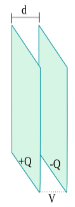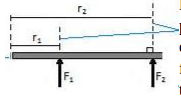## Parallel plate capacitor

### Definition of the parallel plate capacitorThe parallel plate capacitor is a capacitor that consists of two parallel conductor plates, each plate having an equal cross-sectional area (A) and two plates separated by a certain distance (d), as shown in the figure left. One of the conductor plates is positively charged (+Q) while the other conductor plate is negatively charged (-Q), where the amount of electric charge on each plate is equal. So that the charge does not move to the air molecule, the capacitor is isolated from the environment, and between the two plates, there is a vacuum.

## Kepler’s law

Do you still remember the memories of first riding a car? When in a moving car, you see as if a tree or building is moving. At that time, you might think the trees or buildings are moving, while you and the car are in rest. In fact, you and the car move, while the trees or buildings are rest. This experience of fake motion is actually experienced every day. Every morning, “sunrises” on the eastern horizon then move west and “sets” on the western horizon in the afternoon.

Likewise, at night, you often see the moon moving from east to west. Have you ever thought or guessed that the sun and moon moved around the earth, while the earth was in rest?

## Moment of force

### Article about Moment of force

#### 1. Lever arm

Review an object that rotates, such as the door of a room. When the door is opened or closed, the door rotates. The hinges that connect the door to the wall act as the axis of rotation.The door image is seen from above. Review an example where the door is pushed in the same two forces that have the same magnitude and direction, where the direction of the force is perpendicular to the door. At first, the door is pushed with a force of F1, r1 from the axis of rotation. Subsequently, the door is pushed with the force of F2, r2 away from the axis of rotation. Although the magnitude and direction of the force F1 = F2, the force of F2 causes the door to rotate faster than the force of F1. In other words, the force of F2 causes a greater angular acceleration compared to the force of F1. You can prove this.

## Newton’s second law on rotational motion

### 4.1 The relationship between the moment of force, the moment of inertia, and the angular acceleration

If there is a resultant force (ΣF) acting on an object with mass (m) then the object moves linearly with a certain acceleration (a). The relationship between the resultant force, mass, and acceleration is expressed by the equation:

ΣF = m a

This is the equation of Newton‘s second law.

The quantities of the rotational motion which are identical to the resultant force (ΣF) in linear motion is the resultant moment of force (Στ). The quantities of the rotational motion that are identical to mass (m) in linear motion is the moment of inertia (I). The quantities of the rotational motion that are identical to acceleration (a) in linear motion is the angular acceleration (α).

## Center of gravity

### 1. Definition of the center of gravity

A rigid body is composed of many particles; therefore, the gravitational force acting on each of these particles. In other words, each particle has its weight. The center of gravity of an object is a point on the object where the weight of all parts of the object is considered to be centered at that point.

## Types of equilibrium of the rigid body

### Article about the Types of equilibrium of the rigid body

Not all things we find in everyday life always rest. Maybe at first the object rest, but if it is moved (for example by the wind) objects can move. The problem is, whether after moving, objects return to their original position or not. This depends on the type of balance of the object. After moving, there will be three possibilities, namely:

(1) the object returns to its original position,

(2) the object moves away from its original position,

(3) the object remains in its new position.

## Equilibrium of a rigid body

### 1. First condition

Newton’s Second Law states that if the resultant force on an object (an object considered as a single particle) is not zero,

then the object will move with constant acceleration, where the direction of the object’s motion = the direction of the total force. If the resultant force is zero, then the object is at rest or moving at a constant speed.

ΣF = m a

When an object is at rest or moves at a constant speed, the object does not have acceleration (a). Because acceleration (a) = 0, the equation above changes to:

## Springs in series and parallel

### 1. Springs in series

If the spring is connected in series, as in the figure on the side, then:

1. The increase in the length of spring = the increase in length 1 + the increase in length 2

Δy = Δy1 + Δy1

2. The force experienced by equivalent spring = the force experienced by spring 1 = the force experienced by spring 2

Fs = F1 = F2

## Hooke’s law

### 1. Hooke’s law for springs

If the spring is pulled to the right, the spring will stretch and increase in length (figure 1). If the pull force is not huge, it is found that the increase in spring length (Δx) is proportional to the magnitude of the pull force (F). In other words, the greater the pull force, the greater the length of the spring. Comparison of the magnitude of the pull force (F) and the increase in the spring length (Δx) is constant.

## Ohm’s law

### Definition of Ohm’s law

In almost all metal conductors, the electric field is proportional to the density of the electric current, where the ratio of the electric field to the electric current density is constant. Mathematically expressed through the equation:

ρ = E / J

E = electric field, ρ = resistivity, J = current density

The constant ρ is called resistivity, whose value is constant and does not depend on the electric field that gives rise to the electric current.